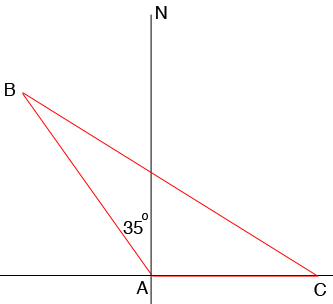SEARCH HOMEMath Central Quandaries & QueriesQuestion from john, a student: two planes leave an airport at the same time, one going northwest (N35*W)at 400 mph and the other going east at 332 mph. How far apart are the planes after 4 hours to the nearest mile?Hi John,

I drew a diagram 4 hours after the planes left with the airport $A$ at the origin, the first plane at $B$ and the second at $C.$What is the distance from $A$ to $C?$ What is the distance from $B$ to $A?$ You want the distance from $C$ to $B.$ The Law of Cosines should help.

PennyMath Central is supported by the University of Regina and the Imperial Oil Foundation.